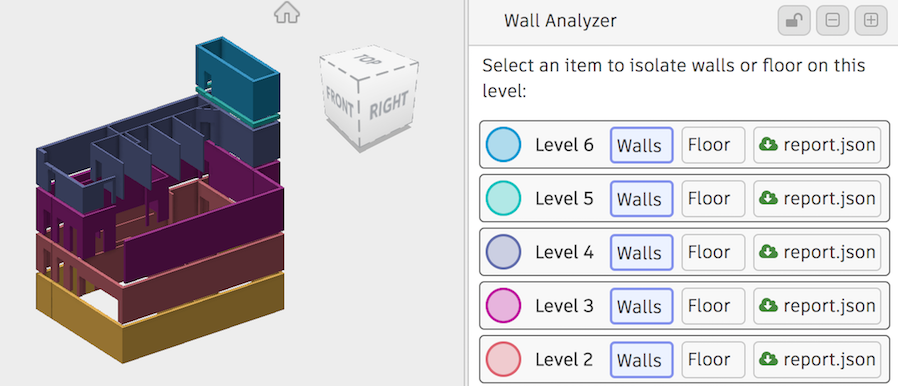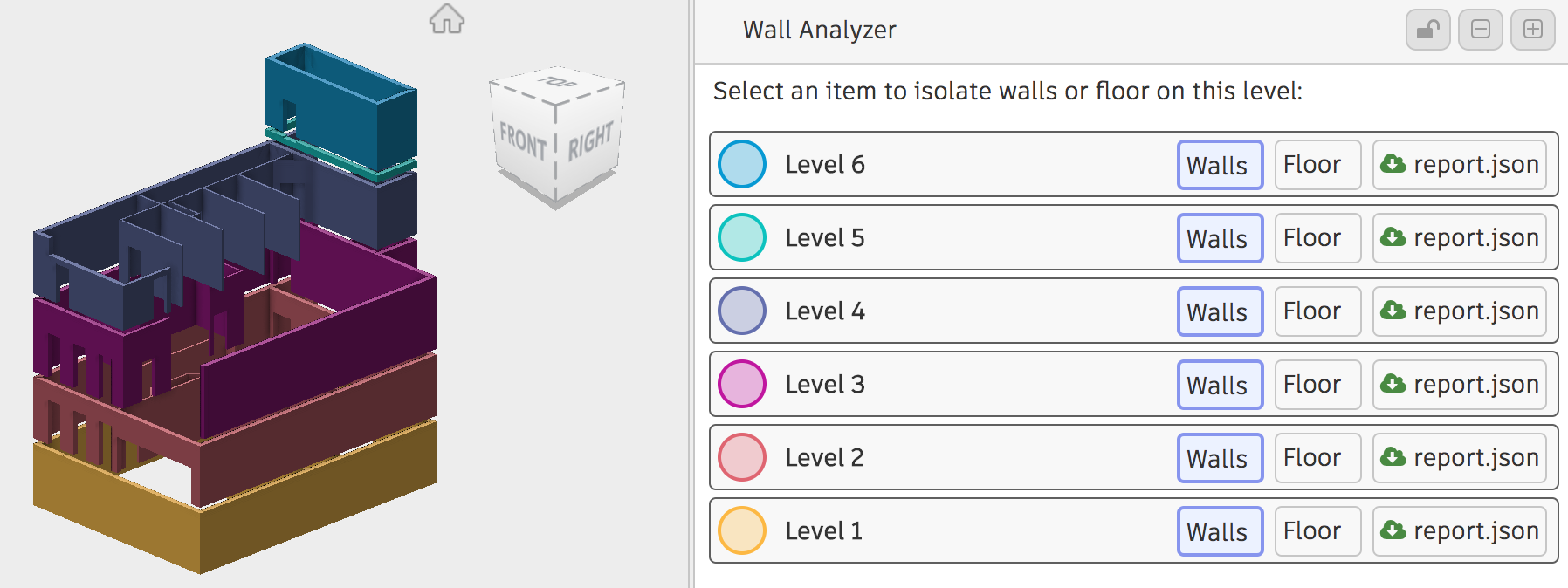14 Jun 2017

# Boolean Operations in the Forge ViewerPhilippe LeefsmaHere is a topic I stumbled across while working on a new sample for a customer: the basic idea is to isolate walls for each level on a building model extracted from a Revit file in the Forge Viewer. The components hierarchy contains "Walls" and "Floors" categories, so it's easy to identify the dbIds we are interested in, however each wall can potentially spans across multiple levels. So my approach was to determine the bounding volume between two consecutive floors, create a mesh out of its bounding box and perform a boolean intersection between each wall, which will give the portion of that wall for this specific level.

After googling a bit I quickly find that CSG would be the correct approach:

Constructive solid geometry (CSG) (formerly called computational binary solid geometry) is a technique used in solid modeling. Constructive solid geometry allows a modeler to create a complex surface or object by usingBoolean operators to combine simpler objects.

Luckily there is a THREE.js plugin using that algorithm: ThreeCSG. You can take a look at the examples which illustrate the three basic boolean operations: union, subtract and intersect. The library takes a standard THREE.Mesh and can create a ThreeBSP out of it that you can use for boolean operations:

``````// basic BSP union example ...

var bsp1 = new ThreeBSP(mesh1)
var bsp2 = new ThreeBSP(mesh2)

var bspUnion = bsp1.union(bsp2)

var result = bspUnion.toMesh(material)``````

However in the Forge Viewer, we are dealing with higher level components, which may be composed of one or several THREE.Mesh and those meshes are not directly compatible with ThreeBSP constructor because the vertices are indexed in an array buffer.

The following code is a complete example that illustrates how to get from a component dbId in the Viewer to a mesh that can be passed to ThreeBSP constructor:

``````/////////////////////////////////////////////////////////
// Creates a standard THREE.Mesh out of a Viewer
// component
//
/////////////////////////////////////////////////////////
buildComponentMesh (dbId, material) {

const vertexArray = []

// first we assume the component dbId is a leaf
// component: ie has no child so contains
// geometry. This util method will return all fragIds
// associated with that specific dbId
const fragIds = Toolkit.getLeafFragIds(
this.viewer.model, dbId)

let matrixWorld = null

fragIds.forEach((fragId) => {

// for each fragId, get the proxy in order to access
// THREE geometry
const renderProxy = this.viewer.impl.getRenderProxy(
this.viewer.model,
fragId)

matrixWorld = matrixWorld ||
renderProxy.matrixWorld

const geometry = renderProxy.geometry

const attributes = geometry.attributes

const positions = geometry.vb
? geometry.vb
: attributes.position.array

const indices = attributes.index.array || geometry.ib

const stride = geometry.vb ? geometry.vbstride : 3

const offsets = [{
count: indices.length,
index: 0,
start: 0
}]

for (var oi = 0, ol = offsets.length; oi < ol; ++oi) {

var start = offsets[oi].start
var count = offsets[oi].count
var index = offsets[oi].index

for (var i = start, il = start + count; i < il; i += 3) {

const a = index + indices[i]
const b = index + indices[i + 1]
const c = index + indices[i + 2]

const vA = new THREE.Vector3()
const vB = new THREE.Vector3()
const vC = new THREE.Vector3()

vA.fromArray(positions, a * stride)
vB.fromArray(positions, b * stride)
vC.fromArray(positions, c * stride)

vertexArray.push(vA)
vertexArray.push(vB)
vertexArray.push(vC)
}
}
})

// builds a standard THREE.Geometry
const geometry = new THREE.Geometry()

for (var i = 0; i < vertexArray.length; i += 3) {

geometry.vertices.push(vertexArray[i])
geometry.vertices.push(vertexArray[i + 1])
geometry.vertices.push(vertexArray[i + 2])

const face = new THREE.Face3(i, i + 1, i + 2)

geometry.faces.push(face)
}

// auto compute normals
geometry.computeFaceNormals()

// creates THREE.Mesh
const mesh = new THREE.Mesh(
geometry, material)

// transform
mesh.applyMatrix(matrixWorld)

// store associated dbId in case we want to invoke
// viewer.model.getProperties (dbId, ...) on that
// mesh
mesh.dbId = dbId

return mesh
}
``````

I initially implemented this logic in my sample, however I quickly found out that when processing all floor and wall meshes of a large building model, the computation could take a long time, hence hanging the browser page. I therefore had to move this computation logic in a worker thread, so the approach is to send each mesh data across, recompose the meshes on the worker side and send back the intersection meshes to the caller page. Below are some of the main snippets of this approach:

``````// send component data from main thread to worker
postComponent (dbId) {

const geometry = this.getComponentGeometry(dbId)

const msg = {
boundingBox: this.getComponentBoundingBox(dbId),
matrixWorld: geometry.matrixWorld,
nbMeshes: geometry.meshes.length,
msgId: 'MSG_ID_COMPONENT',
dbId
}

geometry.meshes.forEach((mesh, idx) => {

msg['positions' + idx] = mesh.positions
msg['indices' + idx] = mesh.indices
msg['stride' + idx] = mesh.stride
})

this.worker.postMessage(msg)
}

getComponentGeometry (dbId) {

const fragIds = Toolkit.getLeafFragIds(
this.viewer.model, dbId)

let matrixWorld = null

const meshes = fragIds.map((fragId) => {

const renderProxy = this.viewer.impl.getRenderProxy(
this.viewer.model,
fragId)

const geometry = renderProxy.geometry

const attributes = geometry.attributes

const positions = geometry.vb
? geometry.vb
: attributes.position.array

const indices = attributes.index.array || geometry.ib

const stride = geometry.vb ? geometry.vbstride : 3

const offsets = geometry.offsets

matrixWorld = matrixWorld ||
renderProxy.matrixWorld.elements

return {
positions,
indices,
offsets,
stride
}
})

return {
matrixWorld,
meshes
}
}

// build the mesh of the worker side
// and generate BSP
function buildComponentMesh (data) {

const vertexArray = []

for (let idx=0; idx < data.nbMeshes; ++idx) {

const meshData = {
positions: data['positions' + idx],
indices: data['indices' + idx],
stride: data['stride' + idx]
}

getMeshGeometry (meshData, vertexArray)
}

const geometry = new THREE.Geometry()

for (var i = 0; i < vertexArray.length; i += 3) {

geometry.vertices.push(vertexArray[i])
geometry.vertices.push(vertexArray[i + 1])
geometry.vertices.push(vertexArray[i + 2])

const face = new THREE.Face3(i, i + 1, i + 2)

geometry.faces.push(face)
}

const matrixWorld = new THREE.Matrix4()

matrixWorld.fromArray(data.matrixWorld)

const mesh = new THREE.Mesh(geometry)

mesh.applyMatrix(matrixWorld)

mesh.boundingBox = data.boundingBox

mesh.bsp = new ThreeBSP(mesh)

mesh.dbId = data.dbId

return mesh
}

function getMeshGeometry (data, vertexArray) {

const offsets = [{
count: data.indices.length,
index: 0,
start: 0}
]

for (var oi = 0, ol = offsets.length; oi < ol; ++oi) {

var start = offsets[oi].start
var count = offsets[oi].count
var index = offsets[oi].index

for (var i = start, il = start + count; i < il; i += 3) {

const a = index + data.indices[i]
const b = index + data.indices[i + 1]
const c = index + data.indices[i + 2]

const vA = new THREE.Vector3()
const vB = new THREE.Vector3()
const vC = new THREE.Vector3()

vA.fromArray(data.positions, a * data.stride)
vB.fromArray(data.positions, b * data.stride)
vC.fromArray(data.positions, c * data.stride)

vertexArray.push(vA)
vertexArray.push(vB)
vertexArray.push(vC)
}
}
}

// send mesh data back to main thread
function postWallMesh (mesh, opts) {

const geometry = mesh.geometry

const msg = Object.assign({}, {
matrixWorld: mesh.matrix.elements,
vertices: geometry.vertices,
floorDbIds: mesh.floorDbIds,
pathEdges: mesh.pathEdges,
msgId: 'MSG_ID_WALL_MESH',
faces: geometry.faces,
dbId: mesh.dbId
}, opts)

self.postMessage(msg)
}``````

Below is how the final sample is looking in the viewer. You can find the complete source code of that demo at: Viewing.Extension.WallAnalyzer

And two live demos, the first one is a rather small model and the second a really big one, so you can appreciate the use of the worker thread:

Wall Analyzer demo - simple office

Wall Analyzer demo - big officeTags:

## Posted By#### Philippe Leefsma

I write JavaScript for the future 3D World Wide Web, A.K.A Forge Platform